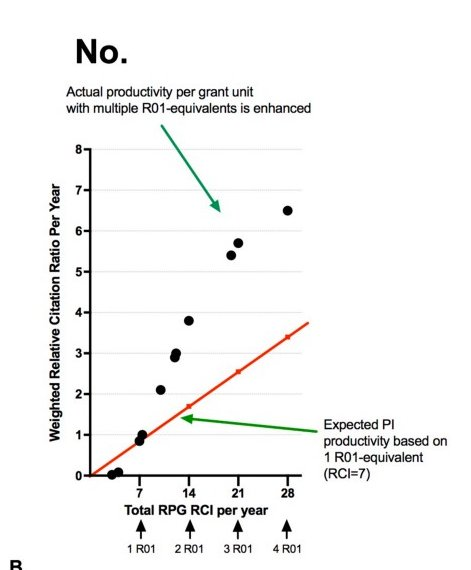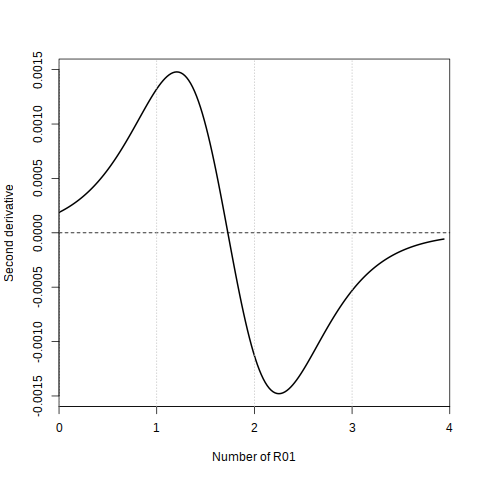# Two grants should be enough

Since I am still waiting for my immune system to win its week-long fight with some viruses (go cytokines go!), I figured I would deviate from the planning and write something related to, not ecology directly, but how to mislead people with statistics. And it involves the logistic curve, so it is basically population dynamics anyways.

The new “Rule of 21” at NIH states, basically, that the investing more than two R01 grants into any scientists is fine, but more than that sees a decrease in productivity. Unsurprisingly, this was met with outrage by some (which is understandable, even though I agree with  the rule), and in the case of the link just before, trying to argument against the rule with bad statistics. And I do not like bad statistics.{: .center-image}

The figure on the left shows the relative citation rate (vertical axis) versus the relative funding in R01 equivalent units (on the horizontal axis). These are the empirical data. Shane Crotty (author of the blog post linked earlier) added a linear regression, forced to go through the origin, to show that returns keep on increasing.

This is wrong.

But let’s start by listing what is right with this linear regression. Both axes are expressed as relative units, so by definition, a PI with the equivalent of a single R01 ($x=7$) will be cited with the equivalent of one R01 ($y=1$). But through any single point, there is approximately an infinite a number of function that can pass, so this is not really informative.

The second point that is assumed to be that a PI with no R01 ($x=0$) will have a relative citation score of 0 ($y=0$. This is called forcing the regression through the origin. Ecologists have argued that even when you have pre-existing knowledge that this should be the case, it is not always advised to force your regression this way. Do we have pre-existing knowledge here? A quick examination of the figure shows that the relative citation rate reaches 0 at about half a R01 equivalent. But we cannot rule out the fact that 0 R01 equivalent would result in 0 citations, so I can live with this hypothesis.

But there is something more problematic in here: using a linear regression at all. This assumes that the rate of increase in citation score is positive, and constant with regard to the equivalent amount of R01; specifically, $y = x/7$. And now is the time to remember that for any problem, there is a trivially wrong null hypothesis that will let you tell the story you want. The lemma, of course, is that this trivially wrong null hypothesis is often a linear regression forced through the origin, but I digress. The bottom line is, this figure is using a blatantly wrong baseline estimation to tell a story (let people get as many R01 as they can).

So what should we do?

The question is to determine if there is a point of inflection around x=14, which is equivalent to 2 R01. A point of inflection, in plain language, is a value for which the function grows slower after than it did before. In terms of citations per R01 invested, this is the number of R01 above which less citations are generated (and therefore the cap for maximal return on investment). If the relationship between y and x is$y=f(x)$, one way to find a point of inflection is to find the value of x for which$f''(x)=0$.$f''$means the second derivative, which represents the rate of change of the rate of change: assuming you are walking,$f(x)$is your position,$f'(x)$is your speed, and$f''(x)$is your acceleration.

Now, instead of setting up a strawman baseline (a linear regression going through 0), we can actually look at the data. And they scream “logistic!”. A logistic function has the shape$f(x) = L/(1+e^{-k(x-x_0})$, where L is the value at  the plateau (the maximum citation score that you can achieve), k is the steepness (the maximum “acceleration” of the citation score when you gain an additional R01), and x0 is the value of the midpoint. Because logistic functions are beautiful, the x0 parameter is (using this expression of the logistic) the solution to$f''(x)=0$, and is therefore the answer we are looking for.{: .center-image}

As I worked with bacterial growth data during my PhD, I am somewhat expert at guesstimating values for these curves. Based on the data, I would start with $6 \leq L \leq 8$, and any positive value for $k$ (about 0.5?). Plugging these values and the logistic function (as well as the data extracted from the figure) in a genetic optimization routine (which is frankly overkill, but I had this code ready to run), I get $x_0 \approx 12.4$. Plotting the value of the second derivative, we get the result on the right. The point of inflection (i) exists and (ii) is reached around approximately two equivalent R01.

The original figure is a teachable moment.

Converting intuition into a numerical framework can work, as long as this is done in a way that is relatable to the data. If not, it becomes easy to mislead or deceive people with what looks like a quantitative argument, but is in fact a misapplication of the methods. This also emphasize how important the visual inspection of the data is before the start of the analysis. There is no way to justify fitting a linear regression through  these data.